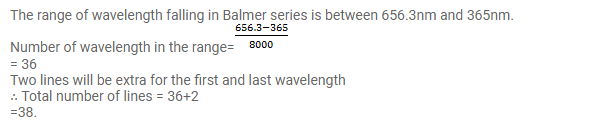# A spectroscopic instrument can resolve two nearby wavelength

Question:

A spectroscopic instrument can resolve two nearby wavelength $\lambda$ and $\lambda+\Delta \lambda$ if $\lambda / \Delta \lambda$ is smaller than 8000 . This is used to study the spectral lines of the Balmer series of hydrogen. Approximately how many lines will be resolved by the instrument?

Solution: